# Knowing that crank AB rotates about point A with a constant angular velocity of 1000 rpm clockwis...

Knowing that crank AB rotates about point A with a constant angular velocity of 1000 rpm clockwise, determine the acceleration of the piston P when θ = 60 .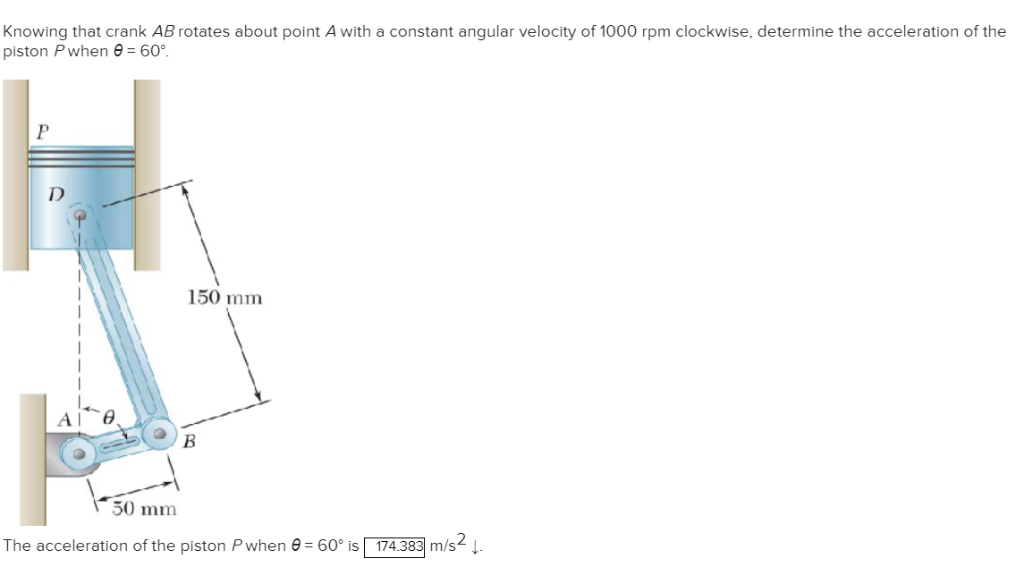Knowing that crank AB rotates about point A with a constant angular velocity of 1000 rpm clockwise, determine the acceleration of the piston P when θ-60° 150 mm 50 mm The acceleration of the piston P when θ 60° is 174383 m/s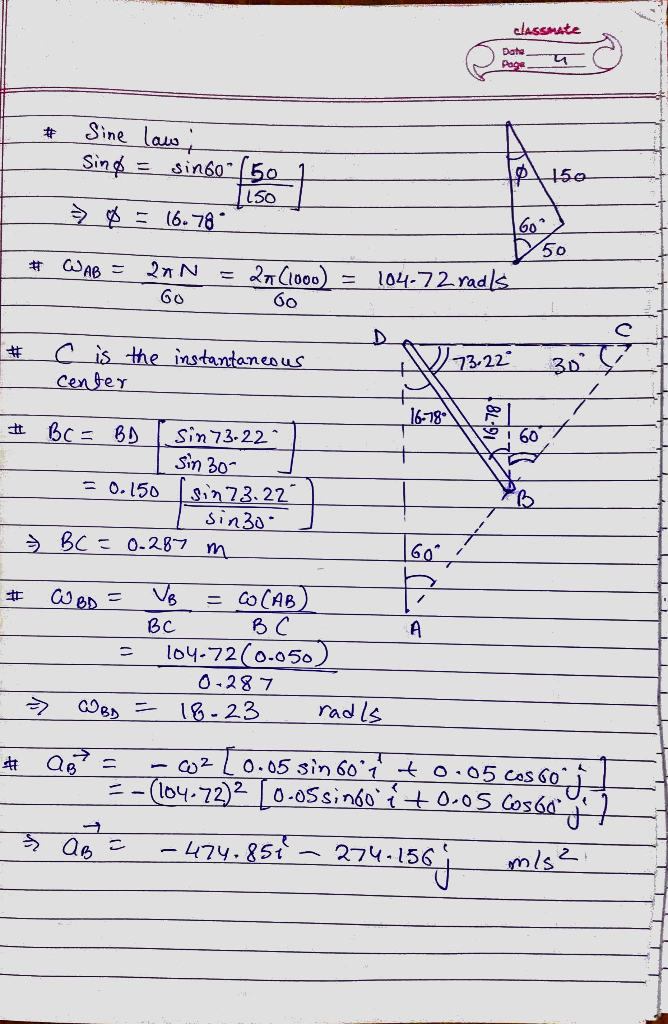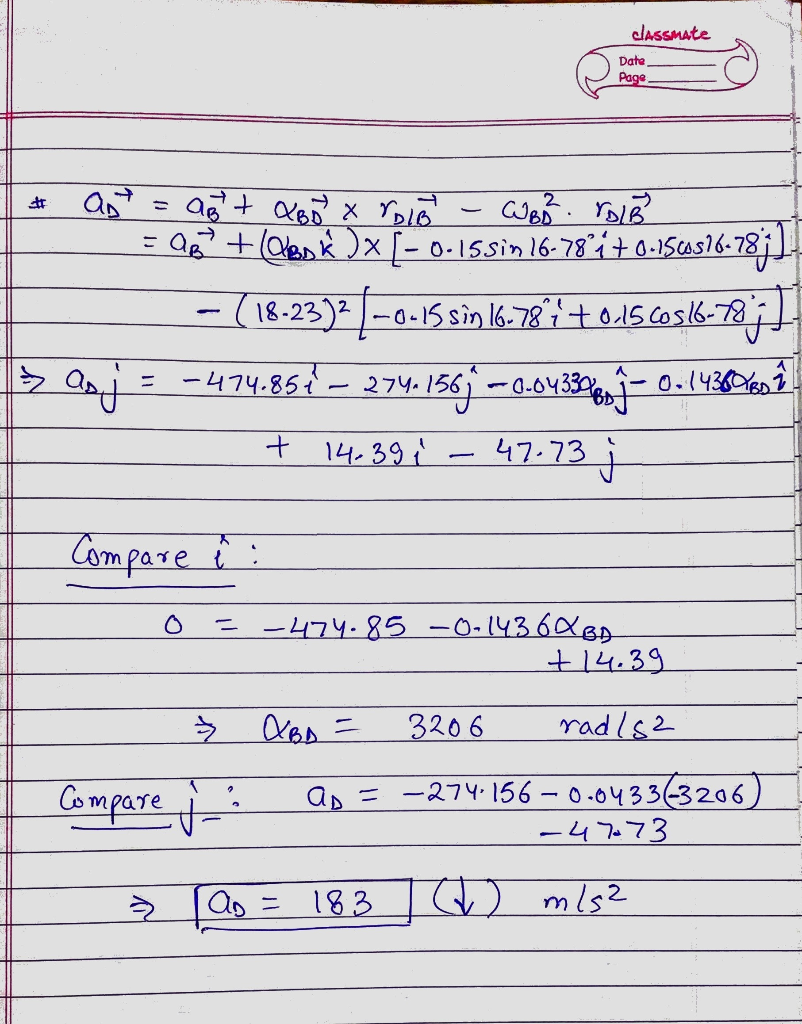##### Add Answer of: Knowing that crank AB rotates about point A with a constant angular velocity of 1000 rpm clockwis...
Similar Homework Help Questions
• ### In the engine system shown, 1 - 160 mm and b 60 mm. Knowing that crank AB rotates with a constant...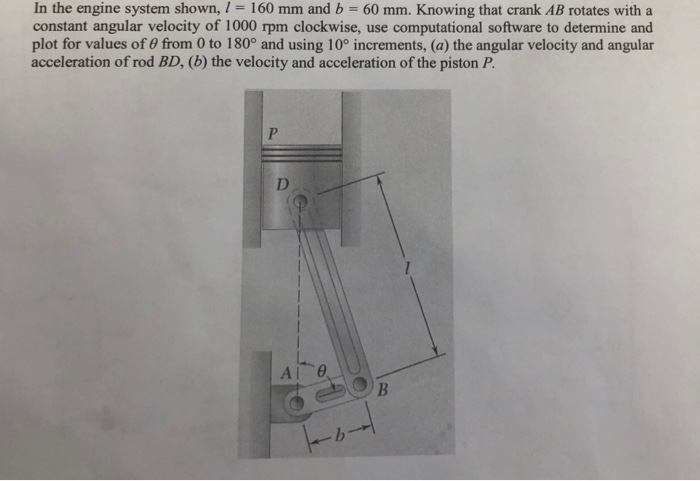can you work it out step by step please. I really need help In the engine system shown, 1 - 160 mm and b 60 mm. Knowing that crank AB rotates with a constant angular velocity of 1000 rpm clockwise, use computational software to determine and plot for values of 0 from 0 to 180° and using 10° increments, (a) the angular velocity and angular acceleration of rod BD, (b) the velocity and acceleration of the piston F. In the...

• ### In the en gine system shown, I -160 mm and b- 60 mm. Knowing that the crank AB rotates with a con...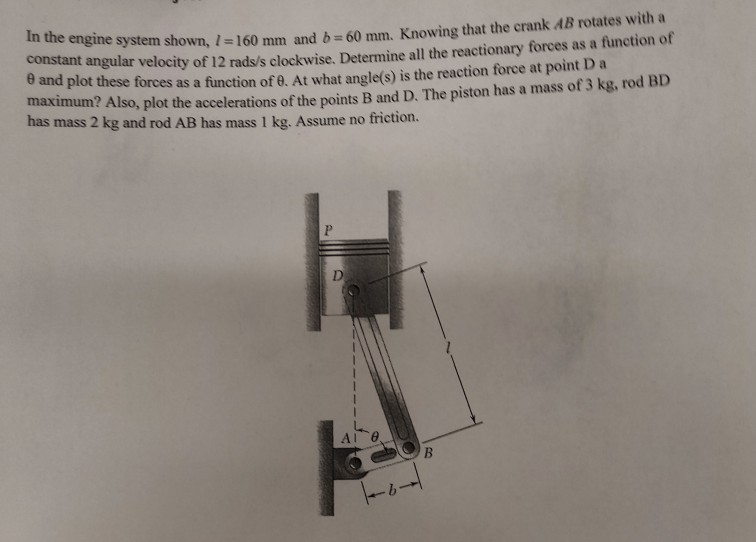In the en gine system shown, I -160 mm and b- 60 mm. Knowing that the crank AB rotates with a constant angular velocity of 12 rads/s clockwise. Determine all the reactionary forces as a function of θ and plot these forces as a function of θ At what angle(s) is the reaction force at point D a maxmum? Also, plot the accelerations of the points B and D. The piston has a mass of3 kg, rod BD has mass...

• ### Dynamics; velocity & angular velocity of piston connecting rodIn the enginesystem shown, l=10in. and b=3in.; the crank AB rotates with a constant angular velocity of 750 rpm clockwise.Determine the velocity of the piston P and the angular velocity of the connecting rod for the position corresponding to θ = 135 degrees.

• ### For the engine system of Prob. 15.C3 of Chap. 15, the masses of piston P and the connecting rod B...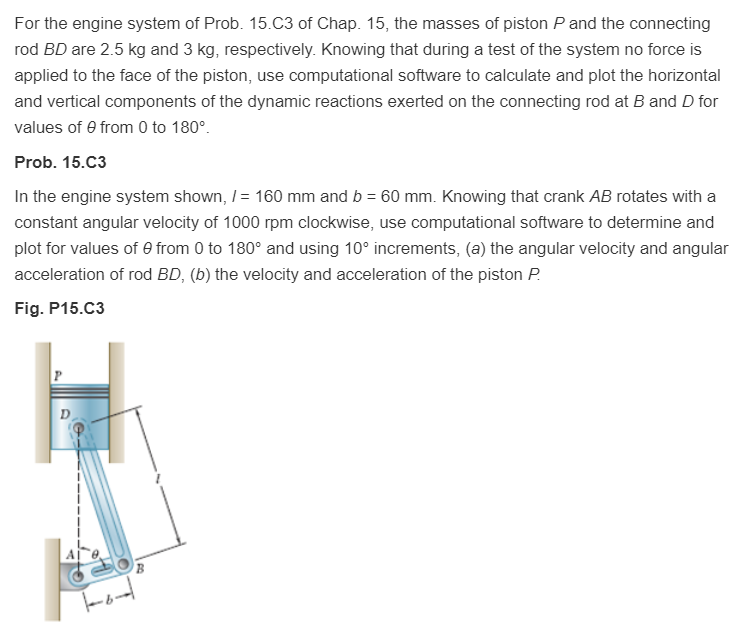For the engine system of Prob. 15.C3 of Chap. 15, the masses of piston P and the connecting rod BD are 2.5 kg and 3 kg, respectively. Knowing that during a test of the system no force is applied to the face of the piston, use computational software to calculate and plot the horizontal and vertical components of the dynamic reactions exerted on the connecting rod at B and D for values of from 0 to 180° Prob. 15.C3 In...

• ### Problem 2 (8 points Knowing that at the instant shown the angular velocity of crank AB is 2.7 rad...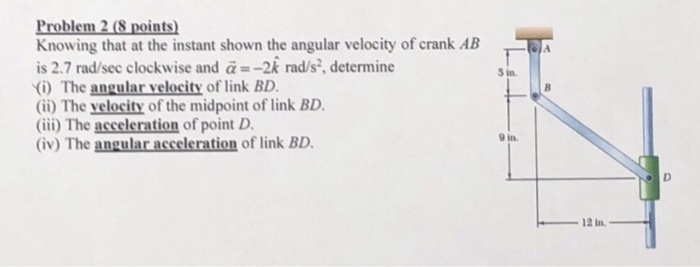Problem 2 (8 points Knowing that at the instant shown the angular velocity of crank AB is 2.7 rad/sec clockwise and α =-2k rad/s, determine i) The angular velocity of link (ii) The velocity of the midpoint of link BD. (iii) The acceleration of point D. (iv) The angular acceleration of link BD 5 in. 9 in. 12 in Problem 2 (8 points Knowing that at the instant shown the angular velocity of crank AB is 2.7 rad/sec clockwise and...

• ### Rod AB of length R = 15 in. rotates about A with a constant clockwise angular velocity. . .Rod AB of length R = 15 in. rotates about A with a constant clockwise angular velocity of 5 rad/s. At the same time, rodBD of length r = 8 in. rotates about B with a constant counterclockwise angular velocity of 3 rad/s with respect to rodAB. Knowing that ,determine for the postion shown the acceleration of point D.Any help will be appreciated!!The answer in the book is given as 392 in./s^2 at angle of 4.05 degrees (4.05 degrees is...

• ### determine velocityIn thepiston/crankshaft/connecting rod system shown in Figure 1, crank ABof length 2 in. rotates about point A with a constant angularvelocity of 1000 rpmcounter-clockwise. Determine:the velocity of the piston P when θ =90o,the acceleration of the piston P when θ =90o.

• ### determine velocityIn thepiston/crankshaft/connecting rod system shown in Figure 1, crank ABof length 2 in. rotates about point A with a constant angularvelocity of 1000 rpmcounter-clockwise. Determine:the velocity of the piston P when θ =90o,the acceleration of the piston P when θ =90o.

• ### 3 FBFDs 3 F ma REM:IA (Piston ,sn't rota-hq) The Dynamies Project In the engine system shown, 1-160 mm and b= 60 mm. Knowing that the crank AB rotates with a constant angular velocity of 12 r...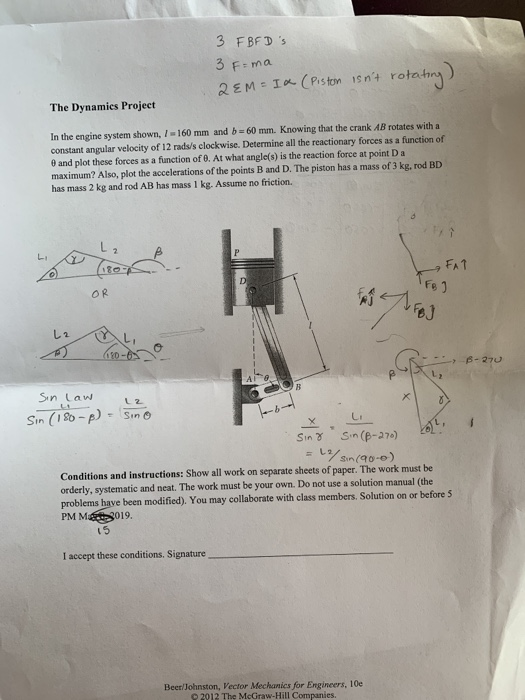3 FBFDs 3 F ma REM:IA (Piston ,sn't rota-hq) The Dynamies Project In the engine system shown, 1-160 mm and b= 60 mm. Knowing that the crank AB rotates with a constant angular velocity of 12 rads/s clockwise. Determine all the reactionary forces as a function of θ and plot these forces as a function oro. At what angle(s) is the reaction force at point D a maximum? Also, plot the accelerations of the points B and D. The piston...

• ### 15.123.) The disk shown has a constant angular velocity of 500 rpm counterclockwise. Knowing that rod...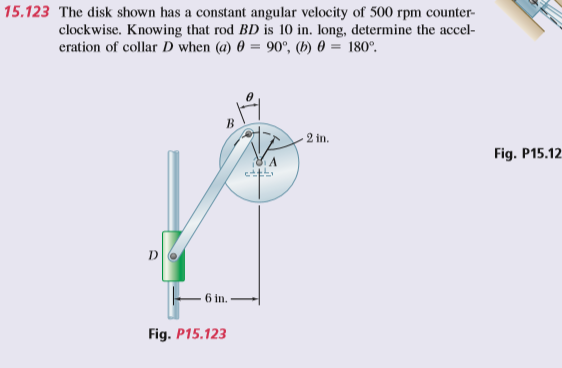15.123.) The disk shown has a constant angular velocity of 500 rpm counterclockwise. Knowing that rod BD is 10 in. long, determine the acceleration of collar D when θ=55°. 15.123 The disk shown has a constant angular velocity of 500 rpm counter- clockwise. Knowing that rod BD is 10 in. long, determine the accel- eration of collar D when (a) 90°, (b) 180 in Fig. P15.12 6 in Fig. P15.123

Need Online Homework Help?